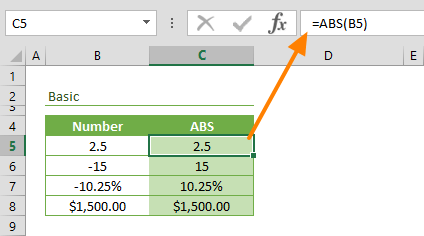The ABS function is a Math formula that returns the absolute value of a given number. The absolute value of a number represents number's distance from zero, regardless of the direction. This means that the distance of a number has the same distance as negative number -x from zero. In this guide, we’re going to show you how to use the absolute value function (ABS) in Excel and also go over some tips and error handling methods.

## Supported versions

• All Excel versions

ABS(number)

## Arguments

 number A real number for which you want the absolute value.

## Examples

### Simple Use Case

The ABS function needs only a single argument named number. The number can be a value, cell reference, or another formula. Below is an example:

=ABS(-15) returns 15### Closest Date

The ABS function can be used to calculate the days between dates. For example, to calculate the difference between a date (MyDate) and today's date, use the following formula:

=ABS(MyDate-TODAY()) returns the difference by days between datesExcel stores dates as numbers. Excel assumes that the date 1/1/1900 is “1″, and increases this number by 1 for each day. For example; 12/31/2018 is equal to 43465.

### Tolerance

Another common usage of the ABS function is to calculate the tolerance. Tolerance represents the acceptable amount a value can change. These amounts are always indicated as positive values. Thus, the ABS function is perfect for these types of calculations. The following formula checks if the absolute value is less than or equal to the allowed tolerance, and returns "Yes" or "No" depending on the outcome.

=IF(ABS(0.5-0.52)<=0.01,"Yes","No") returns "No" because 0.02 > 0.01## Tips

• The ABS function effectively removes the sign of a number and returns a positive value.
• You can use the function to calculate absolute difference between dates.
• The function is useful for tolerance calculations.